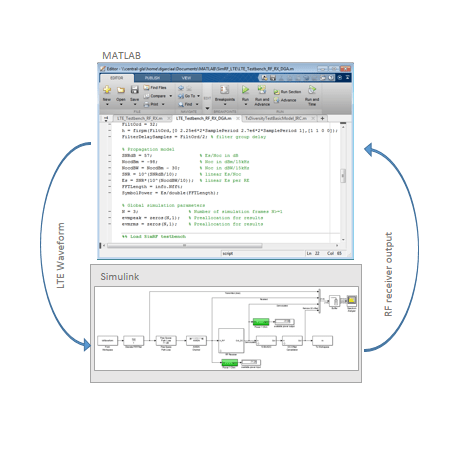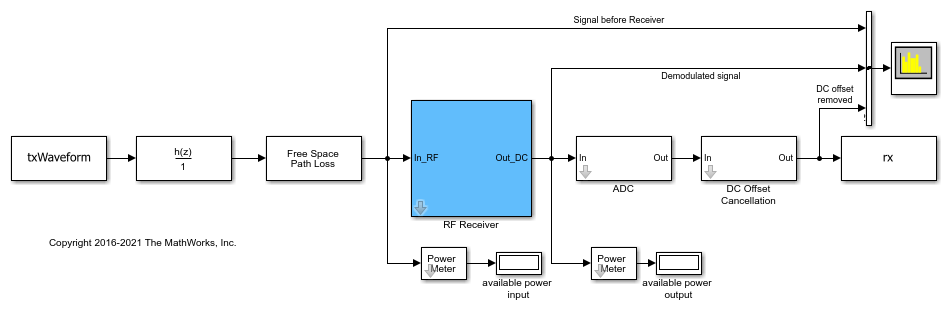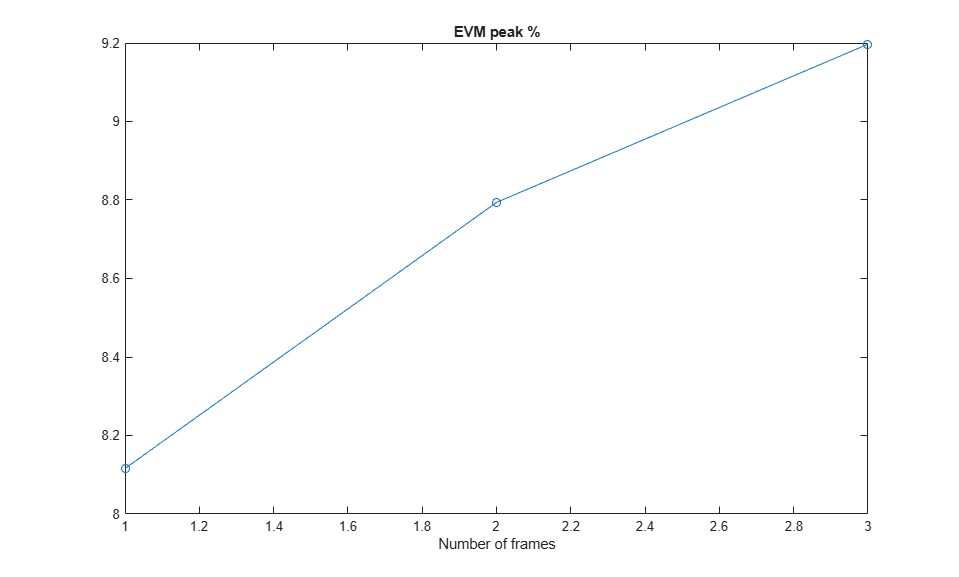# RF Receiver Modeling for LTE Reception

This example demonstrates how to model and test an LTE RF receiver using LTE Toolbox™ and RF Blockset™.

The example requires LTE Toolbox.

### Model Description

The figure below shows the system model for this example. An LTE waveform is generated, filtered, transmitted through a propagation channel and fed into an RF Blockset receiver model. The model can be assembled using commercially available parts. EVM measurements are performed on the RF receiver output.This example is implemented using MATLAB® and Simulink®, which interact at runtime. The functional partition is:The measurement testbench is implemented with a MATLAB script using the Simulink model as the device under test (DUT). LTE frames are streamed between testbench and DUT.

### Generate LTE Waveform

In this section we generate the LTE waveform using the LTE Toolbox. We use the reference measurement channel (RMC) R.6 as defined in TS 36.101 [ 1 ]. This RMC specifies a 25 resource elements (REs) bandwidth, equivalent to 5 MHz. A 64 QAM modulation is used. All REs are allocated. Additionally, OCNG noise is enabled in unused REs.

Only one frame is generated. This frame will then be repeated a number of times to perform the EVM measurements.

```% Configuration TS 36.101 25 REs (5 MHz), 64-QAM, full allocation rmc = lteRMCDL('R.6'); rmc.OCNGPDSCHEnable = 'On'; % Create eNodeB transmission with fixed PDSCH data rng(2); % Fixed random seed (arbitrary) data = randi([0 1], sum(rmc.PDSCH.TrBlkSizes),1); % Generate 1 frame, to be repeated to simulate a total of N frames [tx, ~, info] = lteRMCDLTool(rmc, data); % 1 frame % Calculate the sampling period and the length of the frame. SamplePeriod = 1/info.SamplingRate; FrameLength = length(tx); ```

### Initialize Simulation Components

This section initializes some of the simulation components:

• Band limiting filter: design the filter coefficients, which will be used by the Simulink model. The filter has order 32, with passband frequency equal to 2.25 MHz, and stopband frequency equal to 2.7 MHz.

• Number of frames: this is the number of times the generated frame is repeated

• Preallocate result vectors

```% Band limiting interpolation filter FiltOrd = 32; h = firpm(FiltOrd,[0 2.25e6*2*SamplePeriod 2.7e6*2*SamplePeriod 1],[1 1 0 0]); FilterDelaySamples = FiltOrd/2; % filter group delay % Number of simulation frames N>=1 N = 3; % Preallocate vectors for results for N-1 frames % EVM is not measured in the first frame to avoid transient effects evmpeak = zeros(N-1,1); % Preallocation for results evmrms = zeros(N-1,1); % Preallocation for results ```

This section loads the Simulink model shown below. The model performs the following functions:

• Reading the LTE waveform and the sampling period from the workspace

• Bandlimiting filtering

• Saving results to workspace

The model includes the following components:

• Channel model: includes free space path loss

• RF receiver: includes direct conversion demodulator

• ADC and DC offset cancellation

```% Specify and open Simulink model model = 'simrfV2_lte_receiver'; open_system(model); ```The RF receiver model includes the elements shown belowThis model was initially generated using the RF Budget Analyzer app, and later modified to include additional impairments. Initial RF Budget Analysis of RF Receiver

### Simulate Frames

This section simulates the specified number of frames. This is done in two stages:

• Simulate the first frame

• Simulate the rest of the frames in a loop

The reason for splitting the processing in these two stages is to simplify the code. During the processing of the first frame we need to take into account the delay of the band limiting filter. This is not the case for subsequent frames, since the filter state is maintained between frames. Therefore, the length of the first frame has to be increased slightly to take into account the delay introduced by the filter.

Simulate First LTE Frame

As mentioned for the first simulated frame we need to increase the length of the signal fed to the Simulink model to compensate for the delay introduced by the filter. Next we launch the simulation of the Simulink model without loading any initial state. The model is set to simulate in `Accelerator` mode to decrease run time. After processing the first frame with the Simulink model, its state (`xFinal`) is stored and assigned to `xInitial` for the next time the model is run.

The output of the Simulink model is stored in the variable `rx`, which is available in the workspace. Any delays introduced to this signal are removed after performing synchronization. The EVM is measured on the resulting waveform.

```% Generate test data for RF receiver time = (0:FrameLength+FilterDelaySamples)*SamplePeriod; % Append to the end of the frame enough samples to compensate for the delay % of the filter txWaveform = timeseries([tx; tx(1:FilterDelaySamples+1)],time); % Simulate RF Blockset model of RF RX set_param(model, 'LoadInitialState', 'off'); sim(model, time(end)); % Save the final sate of the model in xInitial for next frame processing xInitial = xFinal; % Synchronize to received waveform Offset = lteDLFrameOffset(rmc,squeeze(rx),'TestEVM'); % In this case Offset = FilterDelaySamples therefore the following % frames do not require synchronization ```

Simulate Successive LTE Frames

Now the rest of the frames can be simulated. First, the model state is set using the value stored in `xInitial` at the output of the previous iteration.

```% Load state after execution of previous frame. Since we are repeating the % same frame the model state will be the same after every frame execution. set_param(model, 'LoadInitialState', 'on', 'InitialState','xInitial'); % Modify input vector to take into account the delay of the bandlimiting % filter RepeatFrame = [tx(FilterDelaySamples+1:end); tx(1:FilterDelaySamples+1)]; EVMalg.EnablePlotting = 'Off'; cec.PilotAverage = 'TestEVM'; for n = 2:N % for all remaining frames % Generate data time = ((n-1)*FrameLength+(0:FrameLength)+FilterDelaySamples) * ... SamplePeriod; txWaveform = timeseries(RepeatFrame,time); % Execute Simulink RF Blockset testbench sim(model, time(end)); xInitial = xFinal; % Save model state % Compute and display EVM measurements evmmeas = simrfV2_lte_receiver_evm_cal(rmc,cec,squeeze(rx),EVMalg); evmpeak(n-1) = evmmeas.Peak; evmrms(n-1) = evmmeas.RMS; end ```
```Low edge EVM, subframe 0: 3.165% High edge EVM, subframe 0: 3.173% Low edge EVM, subframe 1: 3.024% High edge EVM, subframe 1: 3.036% Low edge EVM, subframe 2: 3.004% High edge EVM, subframe 2: 2.992% Low edge EVM, subframe 3: 2.964% High edge EVM, subframe 3: 2.927% Low edge EVM, subframe 4: 3.003% High edge EVM, subframe 4: 3.012% Low edge EVM, subframe 6: 3.001% High edge EVM, subframe 6: 2.983% Low edge EVM, subframe 7: 3.005% High edge EVM, subframe 7: 3.002% Low edge EVM, subframe 8: 3.019% High edge EVM, subframe 8: 3.002% Low edge EVM, subframe 9: 3.050% High edge EVM, subframe 9: 3.041% Averaged low edge EVM, frame 0: 3.024% Averaged high edge EVM, frame 0: 3.017% Averaged EVM frame 0: 3.024% Averaged overall EVM: 3.024% Low edge EVM, subframe 0: 3.165% High edge EVM, subframe 0: 3.173% Low edge EVM, subframe 1: 3.024% High edge EVM, subframe 1: 3.036% Low edge EVM, subframe 2: 3.004% High edge EVM, subframe 2: 2.992% Low edge EVM, subframe 3: 2.964% High edge EVM, subframe 3: 2.927% Low edge EVM, subframe 4: 3.003% High edge EVM, subframe 4: 3.012% Low edge EVM, subframe 6: 3.001% High edge EVM, subframe 6: 2.983% Low edge EVM, subframe 7: 3.005% High edge EVM, subframe 7: 3.003% Low edge EVM, subframe 8: 3.019% High edge EVM, subframe 8: 3.002% Low edge EVM, subframe 9: 3.050% High edge EVM, subframe 9: 3.041% Averaged low edge EVM, frame 0: 3.024% Averaged high edge EVM, frame 0: 3.017% Averaged EVM frame 0: 3.024% Averaged overall EVM: 3.024% ```

### Visualize Measured EVM

This section plots the measured peak and RMS EVM for each simulated frame.

```hf(1) = figure; plot((2:N), 100*evmpeak,'o-') title('EVM peak %'); xlabel('Number of frames'); hf(2) = figure; plot((2:N), 100*evmrms,'o-'); title('EVM RMS %'); xlabel('Number of frames'); ```### Cleaning Up

Close the Simulink model and remove the generated files.

```bdclose(model); close(hf) clear 'hf' ```

### Appendix

This example uses the following helper function:

### Selected Bibliography

1. 3GPP TS 36.101 "User Equipment (UE) radio transmission and reception"